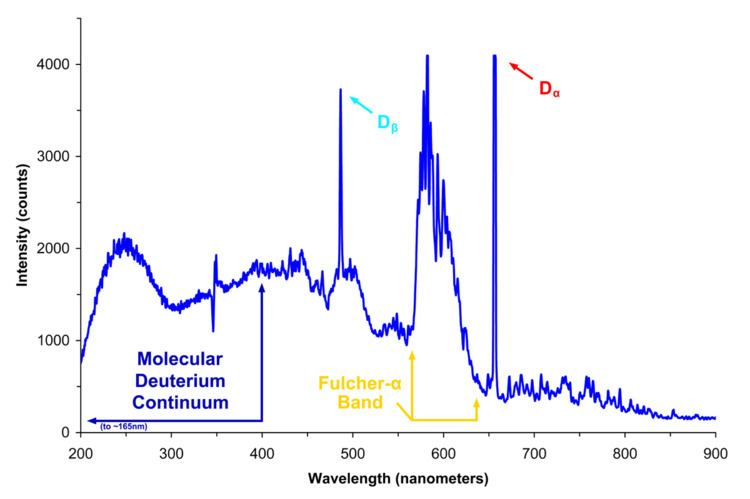# Continuous spectrum

Updated on
Edit
Like
CommentIn physics, a continuous spectrum usually means a set of attainable values for some physical quantity (such as energy or wavelength) that is best described as an interval of real numbers. It is opposed to discrete spectrum, a set of attainable values that is discrete in the mathematical sense, where there is a positive gap between each value and the next one.

The classical example of a continuous spectrum, from which the name is derived, is the part of the spectrum of the light emitted by excited atoms of hydrogen that is due to free electrons becoming bound to a hydrogen ion and emitting photons, which are smoothly spread over a wide range of wavelengths; in contrast to the discrete lines due to electrons falling from some bound quantum state to a state of lower energy.

As in that classical example, the term is most often used when the range of values of a physical quantity may have both a continuous and a discrete part, whether at the same time or in different situations. In quantum systems, continuous spectra (as in bremsstrahlung and thermal radiation) are usually associated with free particles, such as atoms in a gas, electrons in an electron beam, or conduction band electrons in a metal. In particular, the position and momentum of a free particle have a continuous spectrum, but when the particle is confined to a limited space its spectrum becomes discrete.

Often a continuous spectrum may be just a convenient model for a discrete spectrum whose values are too close to be distinguished, as in the phonons in a crystal.

The continuous and discrete spectra of physical systems can be modeled in functional analysis as different parts in the decomposition of the spectrum of a linear operator acting on a function space, such as the Hamiltonian operator.

Continuous spectrum Wikipedia